# Motion and Time NCERT Solutions - Class 7 Science

## NCERT Solutions for Class 7 Science Chapter 13 - Free PDF Download

NCERT Solutions for class 7 science chapter 13 PDF is finally available for all the students on the official website of Vedantu. You can download the solution PDF at any time as per your convenience. It provides you with all the accurate solutions of NCERT questions. All these solutions are collectively prepared by the Vedantu science tutors who have long years of teaching experience. NCERT solutions will help you gain a better knowledge of the chapter. You can also download NCERT Solutions for Class 7 Maths to help you to revise complete syllabus and score more marks in your examinations.

In case you have any doubts about this topic, you can reach out to Vedantu experts through our official website and they will help you clear all the doubts.

Topics Covered in NCERT Solutions Class 7 Science Chapter 13: Motion and Time

The following topics are discussed in Chapter 13 of Class 7 Science.

• Introduction to motion

• Introduction to the concept of distance and displacement

• Introduction to speed

• Measurement of time

• Units of time and speed

• Calculating time and speed

• Distance-time graph

Do you need help with your Homework? Are you preparing for Exams?
Study without Internet (Offline)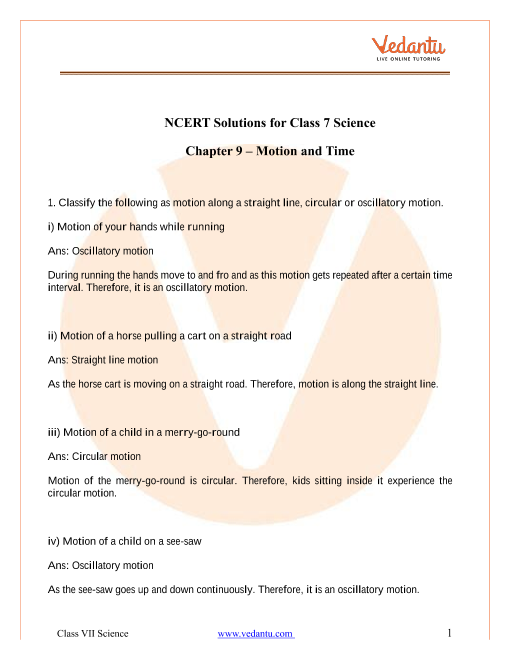## Access NCERT Solutions for Class 7 Science Chapter 13 – Motion and Time

1. Classify the following as motion along a straight line, circular or oscillatory motion.

i) Motion of your hands while running

Ans: Oscillatory motion

During running the hands move to and fro and as this motion gets repeated after a certain time interval. Therefore it is an oscillatory motion.

ii) Motion of a horse pulling a cart on a straight road

Ans: Straight line motion

As the horse cart is moving on a straight road. Therefore motion is along the straight line.

iii) Motion of a child in a merry-go-round

Ans: Circular motion

Motion of the merry-go-round is circular. Therefore kids sitting inside it experience the circular motion.

iv) Motion of a child on a see-saw

Ans: Oscillatory motion

As the see-saw goes up and down continuously. Therefore it is an oscillatory motion.

v) Motion of the hammer of an electric bell

Ans: Oscillatory motion

When the hammer vibrates the bell it starts vibrating. It is an example of oscillatory motion.

vi) Motion of a train on a Straight Bridge

Ans: Straight line motion

The train is moving on the straight bridge. It exhibits motion of a straight line.

2. Which of the following are not correct?

i) The basic unit of time is second

Ans: Correct

SI unit of time is second.

ii) Every object moves with a constant speed

Ans: Not correct

Speed of the object is constant or variable.

iii) Distances between two cities are measured in kilometers

Ans: Correct

The distance between two cities is very large. And as a kilometer is a bigger unit therefore it is used to measure the distance between two cities.

iv) The time period of a given pendulum is not constant

Ans: Not correct

The time period depends upon the length of the thread. Therefore it will be constant for a particular pendulum.

v) The speed of a train is expressed in m/h

Ans: Not correct

The speed of the train is measured either in km/h or m/s.

3. A simple pendulum takes 32 s to complete 20 oscillations. What is the time-period of the pendulum?

Ans: The time taken to complete one oscillation is known as the time period of the pendulum.

$Time\text{ }Period\text{ }=\text{ }\dfrac{\left( Total\text{ }time\text{ }taken \right)}{\left( Number\text{ }of\text{ }Oscillations \right)}\text{ }$

Given:   $20$ oscillations taking $32s$ to complete.

$\therefore 1\text{ }oscillation\text{ }will\text{ }take\text{ }=\dfrac{\left( 32 \right)}{\left( 20 \right)\text{ }}\text{ }sec\text{ }=\text{ }1.6\text{ }second$

$\therefore \text{ }1.6s$is the time period of the pendulum.

4. The distance between two stations is$\mathbf{240}\text{ }\mathbf{km}$. A train takes$~\mathbf{4}~$hours to cover this distance. Calculate the speed of the train.

Ans: $Speed\text{ }=\text{ }\dfrac{\left( Distance\text{ }travelled \right)}{\left( Time \right)}\text{ }$

$\Rightarrow Speed=\dfrac{\left( 240\text{ }km \right)}{\left( 4h \right)}\text{ }$

$\therefore Speed=60\text{ k}m/h$

5. The odometer of a car reads $\mathbf{57},\mathbf{321}.\mathbf{0}\text{ }\mathbf{km}$when the clock shows the time $\mathbf{8}.\mathbf{30}\text{ }\mathbf{AM}$ . The odometer reading was changed to$\mathbf{57},\mathbf{336}.\mathbf{0}\text{ }\mathbf{km}$. Calculate the speed of the car in km/min during this time. Express the speed in km/h also.

Ans: Initial reading of the odometer of the car at $8:30\text{ }AM\text{ }=57321.0\text{ }km$

Final reading of the odometer of the car $8:50\text{ }AM\text{ }=\text{ }57336.0\text{ }km$

The car starts at $8:30\text{ }AM$and stops at $8:50\text{ }AM.$

$Distance\text{ }covered\text{ }by\text{ }car\text{ }=\text{ }\left( 57336\text{ - }57321 \right)\text{ }km\text{ }=\text{ }15\text{ }km$

Time taken between $08:30\text{ }AM$to $08:50\text{ }AM\text{ }=\text{ }20minutes$ $=\dfrac{20}{60}\text{ }hour$ = $\dfrac{1}{3}\text{ }hour$

So Speed in km/min

$~~~Speed\text{ }=\text{ }\dfrac{\left( Distance\text{ }travelled \right)}{\left( Time \right)}$

$\Rightarrow Speed=\dfrac{\left( 15km \right)}{\left( 20min \right)}$

$\therefore Speed=0.75km/min$

Speed in km/h

$Speed\text{ }=\dfrac{\left( Distance\text{ }travelled \right)}{\left( Time \right)}$

$\Rightarrow Speed=\dfrac{\left( 15km \right)}{\dfrac{1}{3}h}$

$\Rightarrow Speed=\dfrac{\left( 15\times 3 \right)\text{ }km}{\left( 1h \right)}$

$\therefore Speed=45km/h$

6. Salma takes $\mathbf{15}$minutes from her house to reach her school on bicycle. If the bicycle has a speed of$\mathbf{2}\text{ }\mathbf{m}/\mathbf{s}$, calculate the distance between her house and the school.

Ans : $Speed\text{ }=\text{ }2m/s$

$Time\text{ }taken\text{ }to\text{ }reach\text{ }school\text{ }=\text{ }15\text{ }min$

$\Rightarrow 15\times 60s=900\text{ }s$

$Distance=\left( Speed\text{ }x\text{ }Time \right)$

$\Rightarrow Dis\tan ce=2\times 900=1800m$

Also $1km=\text{ }1000m$

$\therefore Dis\tan ce=1800\times \dfrac{1}{1000}=1.8km.$

The distance between her house and the school is $1.8\text{ }km.$

7. Show the shape of the distance-time graph for the motion in the following cases:

i) A car moving with a constant speed.

Ans: A car moving with a constant speed covers equal distance in equal intervals of time. It will be a uniform motion.

Distance-time graph will be as below: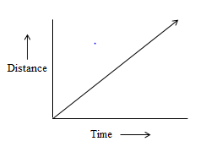ii) A car parked on a side road.

Ans: A car parked on a road there is no change in the distance with the time. No motion.

Therefore the graph obtained will be parallel to x-axis.

Distance- time graph will be as below:-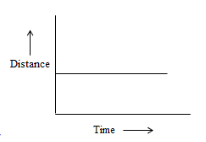8. Which of the following relations is correct?

1. $Speed\text{ }=\text{ }\left( Distance\times Time \right)$

2. $Speed\text{ }=\text{ }\left( \dfrac{Distance}{Time} \right)$

3. $Speed\text{ }=\text{ }\left( \dfrac{Time}{Distance} \right)$

4. $Speed\text{ }=\text{ }\left( \dfrac{1}{\left( Distance\times Time \right)} \right)$

Ans: Speed of an object is given by the relation:-

$Speed\text{ }=\text{ }\left( \dfrac{Distance}{Time} \right)$

9. The basic unit of speed is:

1. $k\mathbf{m}/\mathbf{min}$

2. $\mathbf{m}/\mathbf{min}$

3. $~\mathbf{km}/\mathbf{h}$

4. $\mathbf{m}/\mathbf{s}$

Ans: $\mathbf{m}/\mathbf{s}$.

The unit of distance is meter (m) and of time is second(s).

$Speed\text{ }=\text{ }\left( \dfrac{Distance}{Time} \right)$

Therefore the basic unit of speed is m/s.

10. A car moves with a speed of $\mathbf{40}\text{ }\mathbf{km}/\mathbf{h}$ for $15$ minutes and then with a speed of $\mathbf{60}\text{ }\mathbf{km}/\mathbf{h}$ for the next 15 minutes. The total  distance covered by the car is:

1. $\mathbf{100}\text{ }\mathbf{km}$

2. $\mathbf{25}\text{ }\mathbf{km}$

3. $~\mathbf{15}\text{ }\mathbf{km}$

4. $\mathbf{10}\text{ }\mathbf{km}$

Ans:  25 km

Case 1:

$Speed\text{ }=\text{ }40\text{ }km/h$

$Time=15\text{ }min=\left( \dfrac{15}{60} \right)\text{ }hour$

$Distance\text{ }\left( {{d}_{1}} \right)=Speed\times Time=40\times \left( \dfrac{15}{60} \right)=10\text{ }km$

Case 2:

$Speed=60\text{ }km/h$

$Time=15\text{ }min=\left( \dfrac{15}{60} \right)\text{ }hour$

$Distance\text{ }\left( {{d}_{2}} \right)=\left( Speed\times Time \right)=60\times \left( \dfrac{15}{60} \right)=15\text{ }km$

$Total\text{ }distance\text{ }\left( d \right)=\left( {{d}_{1}}~+\text{ }{{d}_{2}} \right)=10\text{ }km+15\text{ }km=25\text{ }km$

Therefore total distance covered by the car $=\text{ }25km.$

11. Suppose the two photographs, shown in Fig. 13.1 and Fig.13.2, had been taken at an interval of 10 seconds. If a distance of 100 meters is shown by 1 cm in these photographs, calculate the speed of the blue car.

Ans: With the help of the scale we will first measure the distance.

Suppose the distance measured is $2cm$ .

So, the distance covered $d\text{ }=2\times 100=2m$. (Because $1m=100cm$).$~Time\text{ }taken\text{ }=\text{ }10seconds.$

$Speed=\left( \dfrac{Distance}{Time} \right)=\left( \dfrac{200m}{10s} \right)=20m/s.$

Therefore the speed of the blue car $=\text{ }20m/s.$

12. Fig. 13.5 shows the distance-time graph for the motion of two vehicles A and B. Which is one of them moving faster?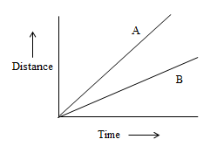Fig. 13.5

Ans: In distance – time graph speed is measured by its slope.

Vehicle A is moving faster as the slope of the graph of A is more than the slope of the graph B.

Therefore Vehicle A is moving faster

13. Which of the following distance time-graphs a truck moving with  speed which is not constant?.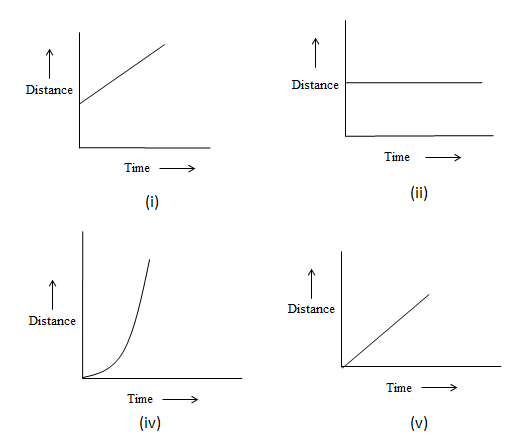Ans: (iii) as the graph is not a straight line, it keeps on changing.

This shows that the truck is moving with variable speed.

### Introduction

You have already studied about different types of motion in class VI. A motion as you know can be along a straight line, circular or periodic. In this chapter, you shall learn in detail about types of motion in detail.

### 13.1 Slow or Fast

You must have seen vehicles moving on the street. Just by observing those vehicles, you can easily identify which one is moving faster than the other. Read activity 13.1 carefully and observe the diagram to understand the concept of slow and fast movement of vehicles in a better way.

### 13.2 Speed

The distance covered by an object in a unit time is known as the speed of the object. You might have heard your father saying that the car is moving at a speed of 60 kilometers per hour. This statement simply means that the car is covering 60 kilometers in one hour. The formula of speed as used in this chapter is given below.

Speed = (Total distance covered)/(Total time taken).

In this section, you shall learn in detail the concept of speed and speed of different objects, in the context of the time taken by the objects to complete any particular task.

## 13.3 Measurement of Time

Have you ever wondered, if the clock wasn’t a part of our lives, how would we have known the time of the day? Well, our ancestors back then obviously did not have clocks with them. You know, many events in nature repeat themselves after specific intervals of time. In this section, you will also learn about a pendulum.

The to and fro motion of a simple pendulum is known as oscillatory motion or periodic motion. The small metallic ball of a pendulum is called the bob. The time taken by a pendulum to complete one oscillation is known as its time period. Observe figure 13.4 (a) and (b) to understand the working of the pendulum in a better manner.

### Units of Time and Speed

As all quantities are measured in metric units, there are units for the measurement of time and speed, as well.

The S.I. unit of time = Second (s).

The S.I. Unit of Speed = Meters per second (m/s).

In this section, you shall learn about the different units of speed.

### 13.4 Measuring Speed

Now, you have learned about the calculation of distance and time. With the time taken by an object to cover a particular distance, you can easily calculate the speed of an object. In this section, you shall learn to calculate the speed of a ball. Similarly, you can use the formula as explained in this to calculate the speed of other objects as well. You must have noticed a meter-like device fitted in your vehicles. It has km/h written in the corner. It is the device that records speed in km/h and is known as a speedometer. The other meter that measures the distance moved by the vehicle is known as an odometer.

### 13.5 Distance - Time Graph

You have already studied about different graphs in mathematics. This section deals with the concept of a distance-time graph. The distance and time can be represented through a bar graph, a pie chart, as well as a line graph. In this section, you shall learn in detail about different distance-time graphs and their formation. Distance-time graphs are generally used to present the motion of any object in a pictorial form. The distance-time graph for the motion of an object that is moving with a constant speed is a straight-line graph. In this section, you will not only learn to read such graphs, but you will also learn the step method of plotting a graph.

Exercise Solutions: 13 Question (2 short questions and 11 long questions).

Extended Learning – Activities and Project (4 Questions).

Key Points Covered in Ncert Solutions Chapter 13 Class 7 Science at a Glance

The following are some of the important points that are discussed in the chapter.

• When an object changes its position with respect to time, it is said to be in motion.

• The speed of the object is the distance an object travels in unit time.

• The speeds of objects allow us to determine which one is travelling faster.

• Metre per second (m/s) is a unit of speed.

• The measurement of time is based on periodic events.

• The motion of objects can be depicted graphically using distance-time graphs.

• For the movement of an item travelling at a steady speed, the distance-time graph is drawn as a straight line.

### Key Features of NCERT Solutions for Class 7 Science

It is always essential to have a proper understanding of all NCERT questions to secure good marks in the exam. With the help of the science solutions prepared by Vedantu experts, students can learn and practice the correct answers to the questions from all topics. It will also help you to develop a better understanding of all the concepts. Some notable key features of Vedantu solution PDF are:

1. It provides easy assistance and important study material to all the students struggling to find accurate answers to their NCERT questions.

2. This PDF is available for free to all the students regardless of any criteria.

3. There is no time restriction for downloading the solution PDF. Students can download it at any time as per their convenience from the official website of Vedantu.

## FAQs on Motion and Time NCERT Solutions - Class 7 Science

1. What is motion and time, according to Chapter 13 of Class 7 Science?

When there is a change in the position of an object with respect to time, it is known as motion. In Class 7 Motion and Time, you will learn about different motions, including why some motions are fast, and others are slow, speed and how it is measured,  how time is measured, and the different units of speed and time. You will also learn how to draw and understand a distance-time graph. You will solve some practical life problems too.

2. What is the unit of Time, according to Chapter 13 of Class 7 Science?

Generally, the basic unit of time, that is second, is used. The bigger units of time are minute and hour. The symbol of the second is s. Though it is the most commonly used unit of time, other units are also used depending on the need. For example, no one tells their age in terms of seconds, minutes or hours, they use year instead of that.

3. What is Speed, according to Chapter 13 of Class 7 Science?

Speed can be referred to as the distance covered by any moving object in unit time. Its basic unit is meter per second (m/s), but the speed of fast-moving vehicles is often expressed in kilometres per hour (km/hr) also. Fast-moving objects have high speed, i.e. they cover more distance in unit time than those of low speed which covers less distance in unit time.

4. What is the unit of speed, according to Chapter 13 of Class 7 Science?

The unit of speed is distance /time, which is meter per second (m/s). Time is, of course, expressed in minutes and hours, and distance can also be expressed in other units like km, cm, mm, etc. Therefore, speed has many other units, like cm/min., km/hr, etc. These units are used only when required.

5. How to get high marks in Chapter 13 of Class 7 Science?

To get high marks in Chapter 13 of Class 7 Science, you need to strategically study the NCERT Science book from the beginning of your session. You should also practice the NCERT solution books to get a better idea of the subject. You can download the NCERT Solution for each chapter in PDF format from Vedantu and read them offline. Practice answer writing too. These solutions are available for free on the Vedantu website and the Vedantu app and are curated by experts.SHARETWEETSHARESUBSCRIBE